Created August 13, 2019

# Light Monitoring System

A monitoring system which helps you to manage the intensity of light in your room in such a smart way.

ExpertFull instructions provided597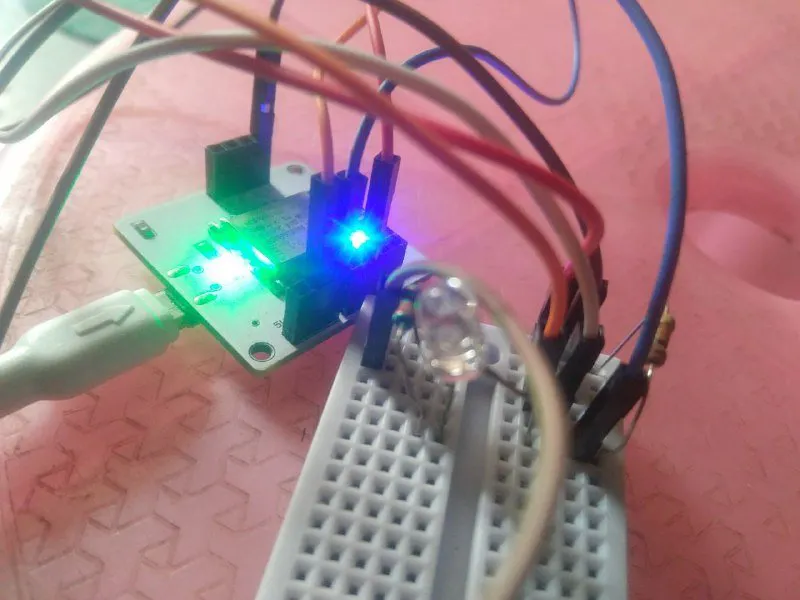## Things used in this project

### Hardware componentsJumper wires (generic)
×1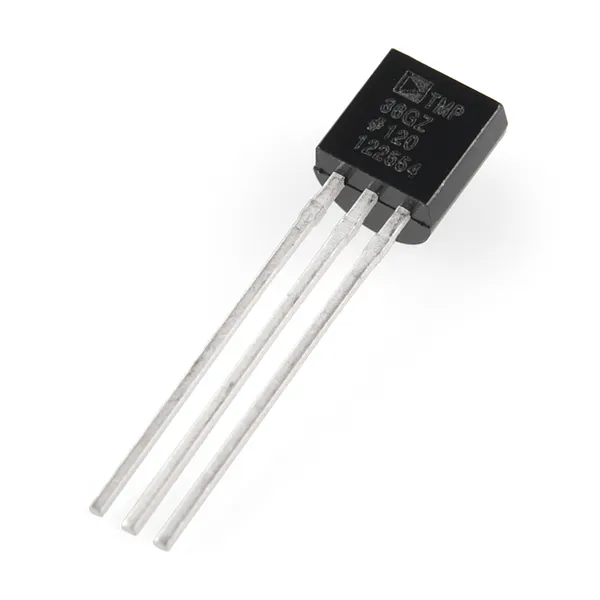Temperature Sensor
×1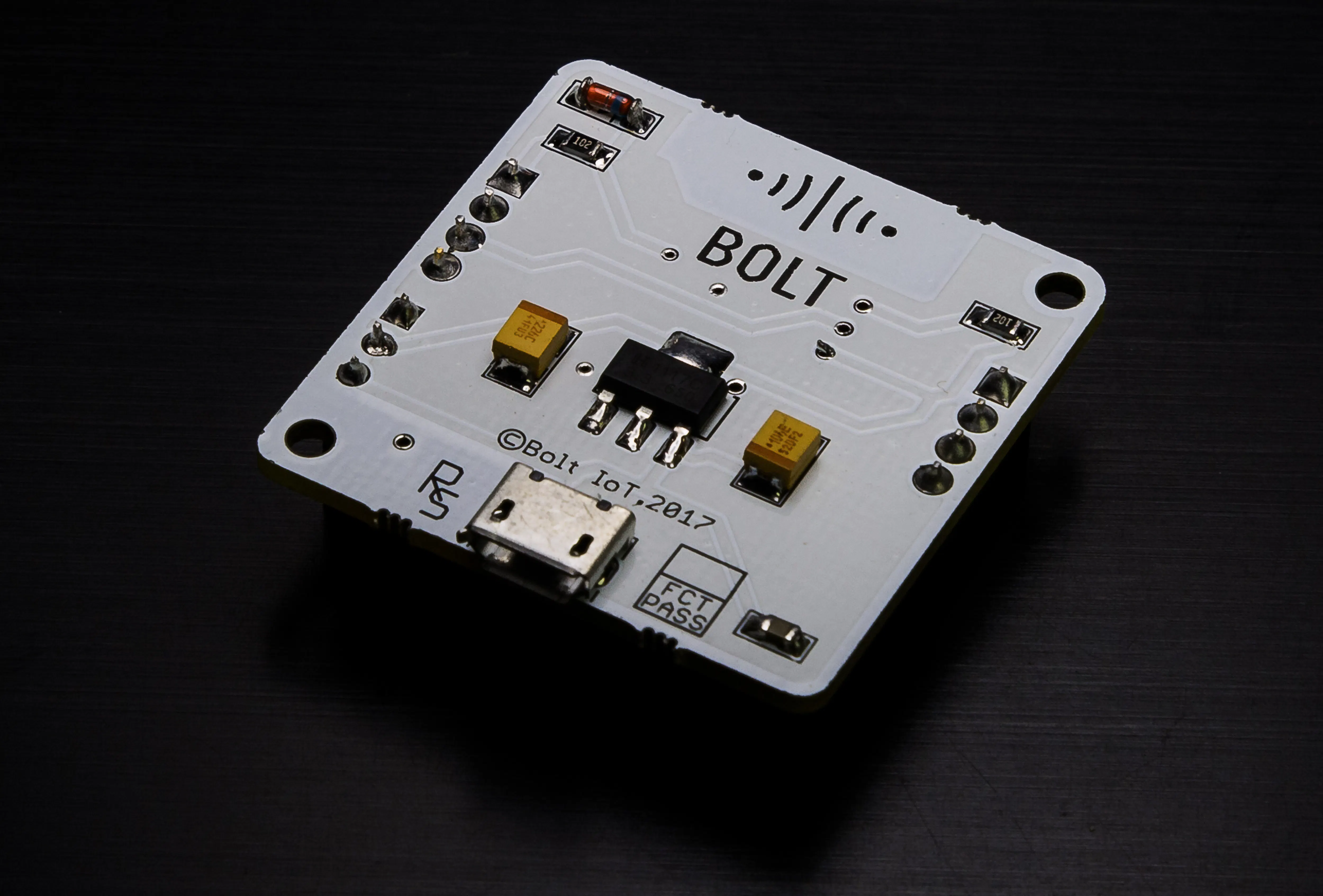Bolt IoT Bolt WiFi Module
×1×1Resistor 10k ohm
×1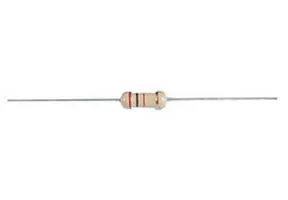Resistor 220 ohm
×1

### Software apps and online services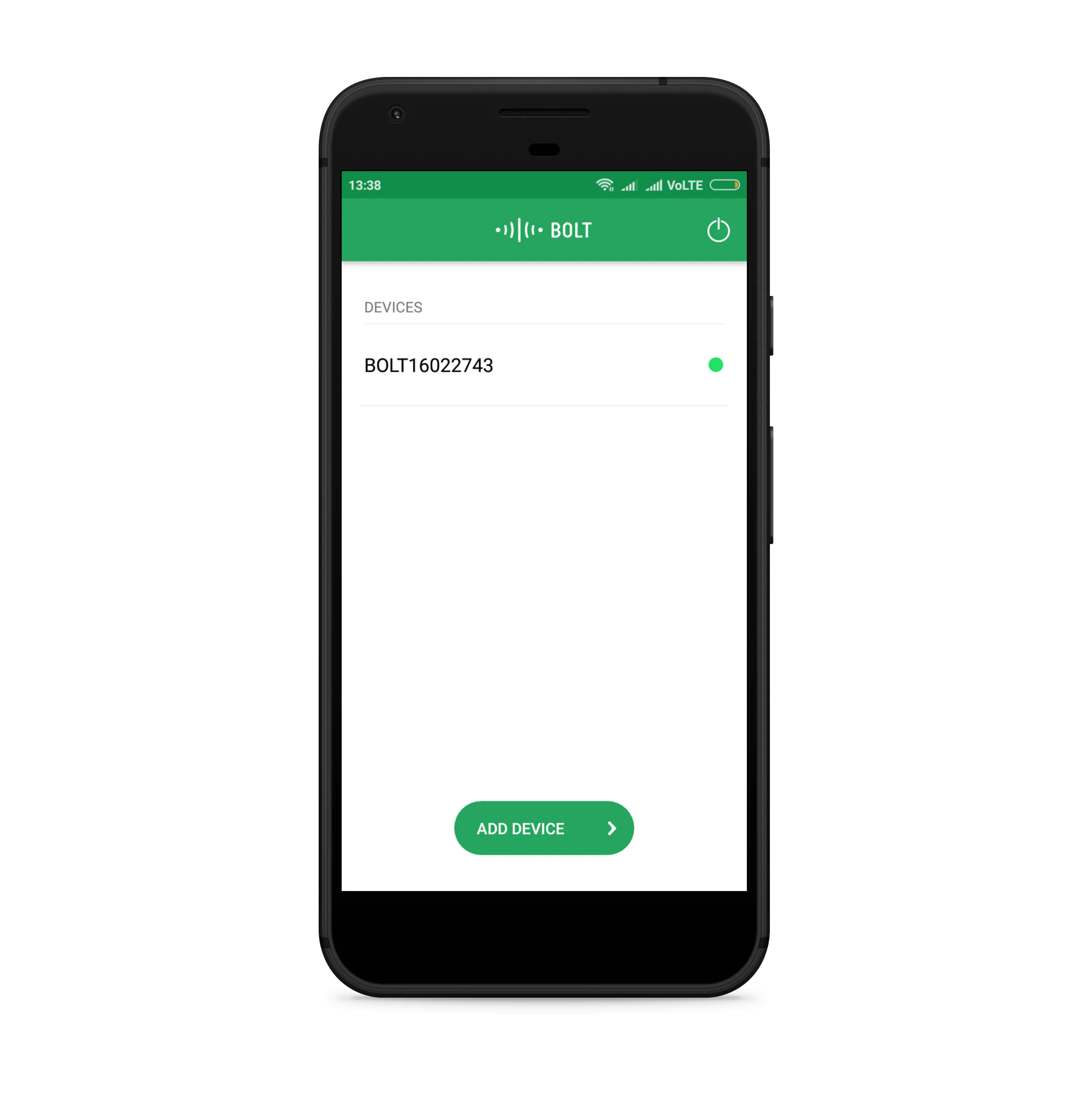Bolt IoT Android AppTwilio SMS Messaging APIBolt IoT Bolt Cloud

## Code

### conf.py

Python
Configuration file
```SID = 'Your_Twilio_SID'
AUTH_TOKEN = 'You_Can_Find_in_your_twilio_control_panel'
FROM_NUMBER = 'YOUR_TWILIO_NUMBER'
TO_NUMBER = '+91YOUR_PHONE_NUMBER'
API_KEY = 'BOLT_API_KEY'
DEVICE_ID = 'BOLT_DEVICE_ID'
FRAME_SIZE = 5
MUL_FACTOR = 3
```

### light_monitoring_system.py

Python
The code of light monitoring system.
```import conf
from boltiot import Sms, Bolt
import json, time, requests

mybolt = Bolt(conf.API_KEY, conf.DEVICE_ID)
"""creating a function to set the value of LED automatically(0-255)
"""
sms = Sms(conf.SID, conf.AUTH_TOKEN, conf.TO_NUMBER, conf.FROM_NUMBER)
"""creating a function to send a message when LDR crosses threshold"""

def set_intensity(pin, value):
mybolt.analogWrite(pin, value)

"""create a function to calculate Required LED Intensity
corresponding to the LDR sensor value
subtract from 255? - if LDR value=1024(sufficient light)-led will be off(0)
if LDR value=0(no light)-led will be on(255)
intermediate value of LDR will corresponds to a specific LED intensity value(0-255)
"""

def converter(sensor_value):
led_intensity= 255-(sensor_value*255/1024)
return led_intensity

while True:
response = mybolt.analogRead('A0') # Read the LDR Sensor Value from A0 Pin
data = json.loads(response)   # Convert value in Json format
print("Sensor value is: " + str(data['value'])) # Print the value
try:
sensor_value = int(data['value'])
print("Calculating required Light Intensity for LED")
led_value_float=converter(sensor_value)  # convert ldr sensor value in required
led_value= int(led_value_float)          # led value in float and then typecast
print(led_value)                         # float into int type
set_intensity('1',led_value)             # this will make LED to glow
# with calculated intensity value
sensor_value = int(data['value'])

if sensor_value > 1024 :
print("Making request to Twilio to send a SMS")
response = sms.send_sms("It's too much bright in here. Switching the light's off. The Current Intensity of light is " +str(sensor_value))
print("Response received from Twilio is: " + str(response))
print("Status of SMS at Twilio is :" + str(response.status))

elif sensor_value < 255 :
print("Making request to Twilio to send a SMS")
response = sms.send_sms("It's too much dark in here. Switching the light's on. The Current Intensity of light is " +str(sensor_value))
print("Response received from Twilio is: " +str(response))
print("Status of SMS at Twilio is :" + str(response.status))

# in case of any error encountered
except Exception as e:
print ("Error occured: Below are the details")
print (e)
time.sleep(3)
```